##### Actions

(diff) ← Older revision | Latest revision (diff) | Newer revision → (diff)

A method of solving a system of linear algebraic equationswhereis a positive-definite (symmetric) matrix. This is at the same time a direct and an iterative method: for any initial approximation, it converges after a finite number of iterations to give the exact solution. In this method the matrix of the system does not change in the process of calculation and at every iteration it is only used to multiply a vector. Therefore, the order of systems that can be solved on computers is high, being determined by the amount of numerical information needed to specify the matrix.

As a direct method, its structure is based on the process of sequential-orthogonalization of a set of vectors, and is an ordinary orthogonalization process (see Orthogonalization method) with respect to the scalar product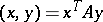. If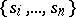is an-orthogonal basis of the space, then for any initial approximation, the exact solution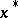of the system can be obtained from the decomposition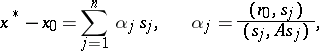where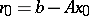is the discrepancy of. In the conjugate-gradient method, the-orthogonal vectors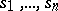are constructed by-orthogonalizing the discrepancies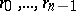of the sequence of approximations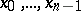, given by the formulasThe vectorsandconstructed in this way have the following properties: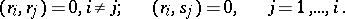(1)

The conjugate-gradient method is now defined by the following recurrence relations (see ):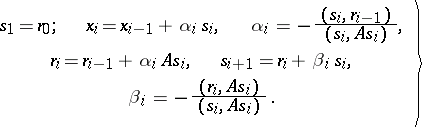(2)

The process ends at some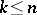for which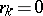. Then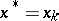. The cut-off point is determined by the initial approximation. It follows from the recurrence relations (2) that the vectors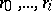are linear combinations of the vectors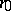,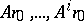. Since the vectorsare orthogonal,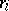can only vanish when the vectors,are linearly dependent, as occurs for example when there are onlynon-zero components in the decomposition ofwith respect to a basis of eigen vectors of. This consideration can influence the choice of initial approximation.

The conjugate-gradient method is related to a class of methods in which for a solution a vector that minimizes some functional is taken. To calculate this vector an iterated sequence is constructed that converges to the minimum point. The sequence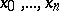in (2) realizes a minimization of the functional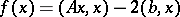. At the-th step of the process (2) the vector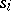coincides with the direction of steepest descent (in gradient) of the surface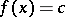on the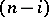-dimensional ellipsoid formed by intersecting the surface with the plane conjugate to the directions.

This method and its analogues have many different names, such as the Lanczos method, the Hestenes method, the Stiefel method, etc. Of all the methods for minimizing a functional, the conjugate-gradient method is best in strategic layout: it gives the maximal minimization aftersteps. However, the calculations (2) under real conditions of machine arithmetic are sensitive to rounding-off errors, and the conditions (1) may be violated. This prevents termination of the process aftersteps. Therefore the method is continued beyonditerations, and it can be regarded as an infinite iterative process for minimizing a functional. Modifications of the calculating scheme (2) that are more resistant to rounding-off errors are known (see , ).

How to Cite This Entry: